#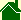Proof Relativity is Illogical

by John T. Nordberg

Most scientific theories are supported inductively. While hundreds of experiments might seem to support a theory, it takes only one argument or experiment to prove a theory wrong. There are hundreds of physical experiments that match the predictions of Relativity. This does not mean Relativity has been "proven" or is a logically correct theory. For example, many of the so-called "proofs" of Relativity also could be described as "proving" the Ball-of-Light Particle Model.

This page provides a real -- rock-solid -- proof that Relativity contains a circular definition that is illogical and unresolvable. Relativity can not withstand this illogical circular definition. An alternative theory is needed to replace Relativity and explain the same observed physical phenomena.

[The alternative to Special Relativity is the Speed of Light Definition of Time. The alternative to General Relativity is the Ball of Light Particle Model. The Ball-of-Light Particle Model describes elementary particles and atomic nuclei as standing spherical waves of electromagnetic energy that create a central-pointing force called gravity. John T. Nordberg]

There are many areas of physics that are heatedly debated. But there is one area that seems fundamental and currently undisputed. That is the area of Basic Units.

[An alternative to SI Basic Units: Nordberg Basic Units.]

Currently, physicists use the seven SI Basic Units. They are:

SI BASIC UNITS
 Quantity Name Symbol 1 length meter m 2 mass kilogram kg 3 time second s 4 electric current Ampere A 5 thermodynamic temperature Kelvin K 6 amount of substance mole mol 7 luminous intensity candela cd

These Basic Units are currently considered the most fundamental concepts in physics. This is critical:

Each SI Basic Unit is a defined concept.

The definitions of these 7 Basic Units are considered fundamental and are the building blocks of modern physics. This is critical:

Basic Units are used to derive
all other concepts in physics.
The other concepts are called Derived Units.

"Force" is one common example of a Derived Unit. The unit of force is called the "Newton" (N = m kg/s2). This Derived Unit derives its meaning from the beginning definitions of the Basic Units: mass, length and time. Mathematically, the "Newton" is a function of: mass, length and time: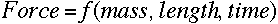Perhaps the simplest Derived Unit -- in currently accepted physics -- is that of "motion." In currently accepted physics, motion is described in terms of "speed" or "velocity." The units for both are: length/time. In other words the concepts "speed" and "velocity" -- in currently accepted physics -- are Derived Units. They derive their meaning from the original definitions of the SI Basic Units: length and time. This is critical:

Mathematically, "speed" and "velocity"
are functions of: length and time: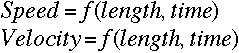Einstein's Special and General theories of Relativity are considered cornerstones for modern physics. The Lorentz transformations -- the key equations central to Relativity -- are usually written in the form: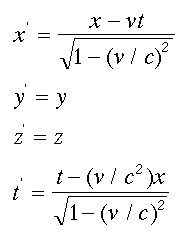This is critical:

The first and fourth equations effectively
redefine the Basic Units: length and time.

However, note there is a Derived Unit -- velocity -- in the denominators of both expressions. This is critical:

Mathematically, these expressions state that:
length is a function of velocity;
and time is a function of velocity: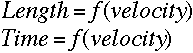Relativity also states that mass is a function of velocity with the expression: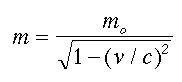Mathematically, this expression effectively states that: mass is a function of length and time: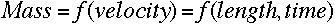## Summary of Logical Argument:

1. SI Basic Units are defined
2. SI Derived Units derive their meaning from the definitions of the SI Basic Units
3. Mass, Length, and Time are 3 of the 7 SI Basic Units
4. Velocity is a SI Derived Unit -- deriving its meaning from the beginning definitions of (SI) Length and (SI) Time
5. Relativity defines (SI) Time as a function of (SI) velocity
6. Relativity defines (SI) Length as a function of (SI) velocity
7. Relativity defines (SI) Mass as a function of (SI) velocity
8. Therefore, (SI) velocity is defined as a function of (SI) time and (SI) time is defined as a function of (SI) velocity.
9. Therefore, (SI) velocity is defined as a function of (SI) length and (SI) length is defined as a function of (SI) velocity.
10. Points 8 & 9 are circular definitions, therefore, using SI Basic Units, Relativity is illogical. This aspect of Relativity is unresolvable. Relativity can not be used as a foundation for physics with this problem.
11. It is also illogical to define one SI Basic Unit -- Mass -- as a function of two other SI Basic Units: Length and Time.

Let me emphasize, I am not questioning the physical effects that have been observed that supposedly support Relativity, or that they are actual phenomena of nature. The point is, Relativity is not a logically correct way to describe these physical observations. Relativity was developed to answer the question of how to add velocities and not exceed the speed of light. Another method of solving this century-old question is needed that does not use the illogical circular definitions within Relativity. The Speed of Light Definition of Time does just that.

To summarize, instead of measuring all motions with our historical standard of motion -- the sun's apparent motion for an earthbound observer as it crosses the sky -- and then correcting this geocentric reference frame for all other observers in other reference frames -- using Relativity -- scientists must redefine time to be the fastest known motion -- the speed of light in free space -- thus allowing one standard of motion to be used in all reference frames without need for a correction factor.